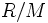# Maximal ideal

This article defines a property of an ideal in a commutative unital ring |View other properties of ideals in commutative unital rings
This property of an ideal in a ring is equivalent to the property of the quotient ring being a/an: field | View other quotient-determined properties of ideals in commutative unital rings

## Definition

### Symbol-free definition

An ideal in a commutative unital ring (or more generally, in any commutative ring) is termed maximal if it is proper (not the whole ring) and it satisfies the following equivalent conditions:

• There is no ideal of the ring properly in between this ideal and the whole ring
• The quotient of the ring by this ideal is a field
• The natural action of$R$ on$R/M$ makes$R/M$ into a simple$R$-module.

### Definition with symbols

An ideal$M$ in a commutative ring$R$ is termed maximal if it satisfies the following equivalent conditions:

• For any ideal$J$ such that$M$$J$$R$,$J$ is equal either to$M$ or to$R$.
• The quotient ring$R/M$ is a field.

## Metaproperties

### Intersection-closedness

This property of ideals in commutative unital rings is not closed under taking arbitrary intersections; in other words, an arbitrary intersection of ideals with this property need not have this property

## Effect of property operators

### The intersection-closure

Applying the intersection-closure to this property gives: intersection of maximal ideals